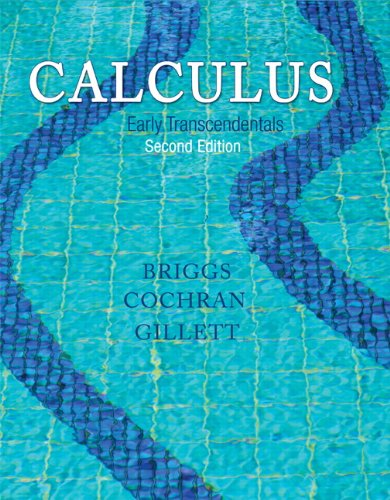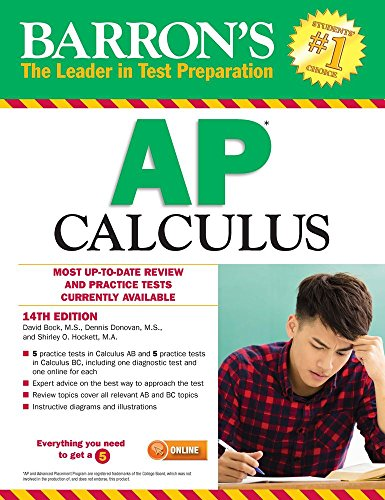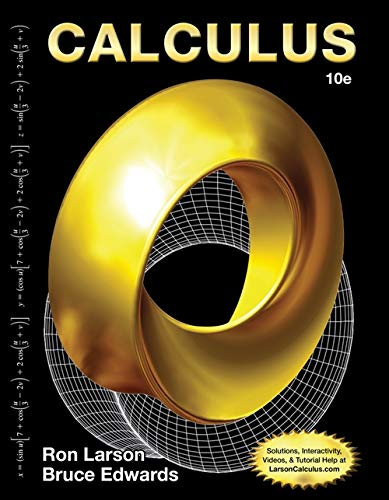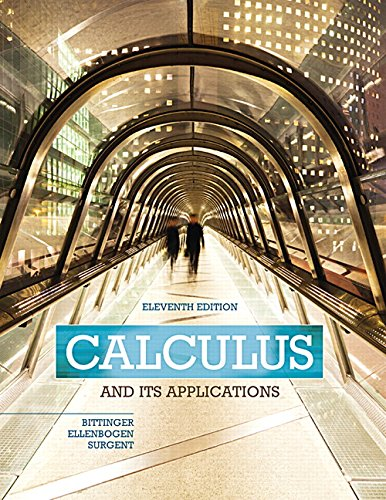# Best Calculus Books for Math Lovers to Enrich their Mathematical Knowledge

One of the beautiful and powerful things about mathematics is the fact that viewing the same concept from multiple perspectives often illuminates the idea. Calculus is a branch of mathematics the purpose of which is to introduce one's mind to the scientific method of analysis. Students who are liberally educated in mathematics should know calculus. Calculus is one of the great intellectual achievements of humankind, and it explains the behavior of moving and changing quantities in our physical world.

Here you will get some books of Calculus if you want to identify areas in mathematics and other fields where Calculus is useful.

## 1. Calculus For Dummies (For Dummies (Math & Science))

Calculus For Dummies (For Dummies( Math & Science)) is the best calculus book for self-study. It's also an excellent book to learn what a student needs to know in understanding Calculus. It provides some additional material in Algebra and Trigonometry as a review in learning the subject. By breaking down differentiation and integration into digestible concepts, this guide helps build a stronger foundation with a solid understanding of the big ideas at work. This user-friendly math book leads you step-by-step through each concept, operation, and solution, explaining the "how" and "why" in plain English instead of math-speak. Through relevant instruction and practical examples, you will soon learn that real-life calculus is not nearly the monster it's made out to be. The writer covers some calculus prerequisites, limits, differentiation, integration, and infinite series. With this comprehensive study guide, you may gain the skills and confidence that make all the difference and may provide a roadmap for success, and the backup you need to get there.

This study material includes:

• Foundations in algebra, trigonometry, and pre-calculus concepts
• Explores sequences, series, and graphing common functions
• Instructs you how to approximate area with integration
• Features things to remember, things to forget, and things you can't get away with
• Excellent reviews of algebra and pre-algebra

## 2. Introductory Calculus For Infants

Are you thinking about how to introduce your little champ with mathematics? Here you go.

Introductory Calculus for Infants is primarily an ABC book using mathematical concepts for each letter. This is the best mathematics books for beginners. It's a  storybook adventure of two friends as they explore the wonders of calculus. It is full of optimism, about friendship, being nice and believing in oneself. At the same time, it familiarizes infants with mathematics in a friendly and happy way. The drawings of this story-book are large, clear and very humorous. The fine colors and stories of this book tend to teach the alphabet more than it teaches math to a child.

## 3. Calculus: Early Transcendentals (2nd Edition)Author: William L. Briggs,Lyle Cochran,Bernard Gillett
Published at: 03/01/2014
ISBN: 0321947347

Calculus: Early Transcendentals (2nd Edition) is a great calculus book for beginners. This much anticipated second edition of the most successful new calculus text published in the last two decades retains the best of the first edition while introducing important advances and refinements.

Authors Briggs, Cochran, and Gillett build from a foundation of meticulously crafted exercise sets, then draw students into the narrative through writing that reflects the voice of the instructor. The examples that are stepped out and thoughtfully annotated, and figures that are designed to teach rather than simply supplement the narrative. The authors appeal to students’ geometric intuition to introduce fundamental concepts, laying a foundation for the development that follows.

This is absolutely an excellent resource which can be used as a classroom text or equally well as a primary reference. It aids with the easy comprehension of calculus by using verbal descriptions and walk through examples.

If you are a calculus lover you would like this book.

## 4. Barron's AP CalculusAuthor: David Bock M.S.,Dennis Donovan M.S.,Shirley O. Hockett Ph.D.
Published at: 01/02/2017
ISBN: 1438008597

Barron’s AP Calculus is absolutely essential for students who are preparing to take either of the Advanced Placement examinations in mathematics. It is aligned with the current exam curriculum and provides comprehensive review and practice exams for both AP Calculus AB and BC. The book has a ridiculous number of practice questions on all of the topics covered in the exam. If you are looking to score well through sheer practice and repetition, then this is the book to buy.

This edition of Barron's AP calculus guide includes:

• Six practice exams in the book: Three for Calculus AB and three for Calculus BC, all modified to reflect the new exam format
• Two online practice exams: One for Calculus AB and one for Calculus BC
• Diagnostic tests help pinpoint strengths and weaknesses so students can focus on areas where they need more study
• Answer explanations for all test questions
• A detailed subject review covering topics for both exams
• Advice to students on efficient use of their graphing calculators
• Concepts and applications of calculus
• Overview on how to use a graphing calculator for mathematical investigations and problem-solving

## 5. Calculus: Early Transcendentals

James Stewart's CALCULUS: EARLY TRANSCENDENTALS is a great study material on advanced calculus. The text of this guidebook is clear, accurate, and filled with relevant, real-world examples. The author conveys not only the utility of calculus to help you develop technical competence, but also gives you an appreciation for the intrinsic beauty of the subject. His patient examples and built-in learning aids will help you build your mathematical confidence and achieve your goals in the course.

The Eighth Edition of  Calculus: Early Transcendentals Provides:

• Concepts and applications of advanced calculus
• Answer explanations for all test questions
• Tools and their uses for enriching calculus
• Conceptual exercises

## 6. CalculusAuthor: Ron Larson,Bruce H. Edwards
Published at: 01/01/2013
ISBN: 1285057090

The Larson CALCULUS, a very comprehensive book that has a long history of innovation in the calculus market. It has been widely praised by a generation of students and professors for its solid and effective pedagogy that addresses the needs of a broad range of teaching and learning styles and environments. Each title is just one component in a comprehensive calculus course program that carefully integrates and coordinates print, media, and technology products for successful teaching and learning. This book has great examples, numerous problems, as well as access to the online website for additional information. It's a great book and highly recommended for anyone studying or reviewing calculus.

This Book Covers:

• Theorems and definitions that provide the conceptual frameworks of calculus
• Historical notes and biographies that provide background information on the foundation of calculus
• Applied exercises and examples with detailed explanations
• Reviews of pre-calculus
• Limits and properties of calculus

## 7. Calculus and Its Applications (11th Edition)Author: Marvin L. Bittinger,David J. Ellenbogen,Scott A. Surgent
Published at: 03/01/2015
ISBN: 0321979397

Calculus and Its Applications is an easy to understand guide to exploring calculus. The book does an excellent job of teaching and giving examples, provides an algebraic review in an appendix, and provides plenty of problems to work out with solutions. Because of its accessible presentation, it can anticipate student needs. It will provide intuitive explanations that work with the carefully crafted artwork to help them visualize new calculus concepts. Additionally, the text’s numerous and up-to-date applications from business, economics, life sciences, and social sciences help motivate students. Algebra diagnostic and review material is available for those who need to strengthen basic skills. Every aspect of this revision is designed to motivate and help students to more readily understand and apply mathematics in real life.

This Edition Provides:

• Reviews of basic algebra
• Definitions and basic concepts of advanced calculus
• Functions and applications of calculus
• Applied exercises and examples with detailed explanations of mathematical terms

## 8. Infinite Powers: How Calculus Reveals the Secrets of the Universe

Infinite Powers: How Calculus Reveals the Secrets of the Universe recounts how calculus tantalized and thrilled its inventors, starting with its first glimmers in ancient Greece and bringing us right up to the discovery of gravitational waves (a phenomenon predicted by calculus). Steven Strogatz has written an outstanding book that presents an overview of Calculus that students, and even professors, often fail to learn. His writing style is both informal and yet precise; the book is very reader-friendly. The content covers 2200 plus years of the history of calculus from Archimedes to the present, and beyond speculating about the future.

Strogatz reveals how this form of math rose to the challenges of each age: how to determine the area of a circle with only sand and a stick; how to explain why Mars goes “backward” sometimes; how to make electricity with magnets; how to ensure your rocket doesn’t miss the moon; how to turn the tide in the fight against AIDS. He also points out the practical applications of calculus.

History shows that calculus is not about complexity; it is about simplicity and proves calculus is truly the language of the universe.

A great read if you like physics or math, or are just curious about why something you have observed acts the way it does.

## 9. Calculus

James Stewart's CALCULUS is an introductory calculus textbook that is clear, illustrious, explicit, and sometimes entertaining allows the student to more simply define the new concept in more ways than one, or the way that best suits them. His patient examples and built-in learning aids will help you build your mathematical confidence and achieve your goals in the course.

This book covers a lot of examples, exercises, and background information. Must have for every student taking a serious calculus course. The explanations are generally clearer than those found in most other calculus texts. If you need a good understanding of the basic concepts of calculus this is the book for you.

## 10. Learn Calculus Quickly: The Complete Guide To Easily Master Calculus in 100 Solved Equations!

Learn Calculus Quickly: The Complete Guide To Easily Master Calculus in 100 Solved Equations! is a complete guide to understanding the basics of calculus along with 100 solved equations. This is the best calculus problem book. It includes the Chain Rule, double integrals, and triple integrals.

This Calculus study guide contains three basic sections:

1. The Limits section covers slope and linear equations, tangent lines, the definition of a limit, and evaluating limit functions.

2. The Derivative section covers differentiation rules for polynomial functions, trigonometric functions, rational functions, exponential functions, radical functions, and natural logarithmic functions. It also includes the Product Rule, the Quotient Rule, and the Chain Rule, first and second derivatives, and partial derivatives.

3. The Integration section covers the integration rules for polynomial functions, trigonometric functions, rational functions, exponential functions, radical functions, and natural logarithmic functions. It has examples of both indefinite and definite integrations.

Thanks for reading this post. If you have any opinion don't hesitate to comment here. Also please subscribe our newsletter to get more updates.